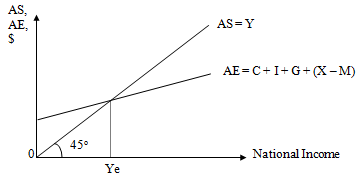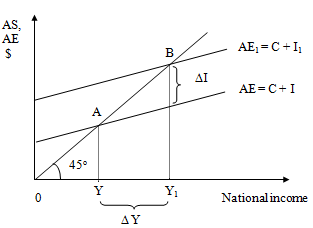#### Keynes Theory of Income and Employment Homework Help

What is the Keynes Theory?

¨      According to Keynes, the equilibrium level of income is determined by the intersection of aggregate expenditure and aggregate supply.

¨      Aggregate supply (AS) is given to be 45o line and Aggregate Expenditure (AE) sloped upward as shown below.¨      The components of AE are :-

1)      Consumption (C)

2)      Investment (I)

3)      Government Expenditure (G)

4)      Net exports (X - M)

¨      Symbolically, it can be written as :-

AE = C + I + G + (X - M)

¨      Among the above components of AE, the most volatile one is Investment expenditure.

Investment Expenditure (I)

¨      Investment refers to any increase in real capital stock of an economy.

¨      It can either be Gross Investment or Net Investment.

Net Investment = Gross Investment - Replacement Investment

Factors Influencing the Level of Investment

a)      Expected Yield/Return Relative to Cost

Cost of investment is represented by the interest rate or the cost of borrowing money while expected yield or return from an investment over its useful life can be expressed as a return on capital cost.

If interest rate is high, then it is no longer profitable to invest.

If businessmen are pessimistic about future, low interest rate will not encourage them to borrow, whereas if they are optimistic, high interest rate will not necessarily discourage them to invest.

c)      The Level of Firm's Profit

A high level of firm's profit appears to stimulate investment because it makes businessmen more optimistic about the future and also they have more funds to invest.

d)     Government Policy

Government may give certain tax incentives to encourage investment (Fiscal Policy).

Monetary Authority may reduce bank rate to effect a reduction of interest rate, hence making borrowing cheaper (Monetary Policy).

The Multiplier and how it Works

¨      Multiplier measures the ratio of the changes in income to a permanent change in the flow of expenditure, that is, it indicates the number of times income increases as a result of a given initial increase in say, investment.

¨      It may be expressed as :-

K =

¨      The size of the multiplier depends on the Marginal Propensity to Consume (MPC).

MPC =

¨      The higher the MPC, the greater will be the multiplier and hence the larger will be the income generated.

Illustration

#### Change in saving1

2

3

4

5

6

7

Subtotal (1-7)

Other periods

Total

100

100.0

75.0

56.3

42.2

31.6

23.7

17.8

346.6

53.4

400.0

75.0

56.3

42.2

31.6

23.7

17.8

13.3

260.0

40.1

300.0

25.0

18.8

14.1

10.5

7.9

5.9

4.4

86.7

13.3

100.0

How an Economy Can Move Towards Equilibrium Income and Full Employment

¨      It is through the multiplier concept that we can assess the magnitude of the changes in National Income caused by a change in investment.

¨      Any increase in investment will have multiplier effect on the income, hence the possibility of achieving a greater level of equilibrium income and employment

This effect can be illustrated in the diagram below